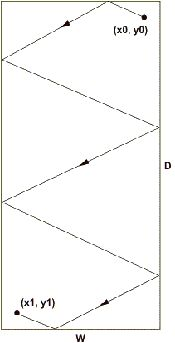# [TimusACM]程序员撞墙的问题

前几日在博客园看到这种在线测试的时候，有一种相见恨晚的感觉，于是随便选了一道感兴趣的题（No.1258：Pool）开始做。为了准确了解题的意思，我把题翻译成中文了，这道题的原理和台球很相似（由于以前常玩可乐8，所以对台球的问题倍感亲切）。但不知道为什么出题人将台球问题说成了一个程序员撞墙的问题。下面是我翻译后的，英语不好，译错的地方请见谅。

## 1258. Pool### 输出

Vasechkin从起点到终点所走的长度，保留小数点后四位。

### 例子

input output
            10 20
9 1
1 19
FLRLRB
52.8015

==============================================================X0,X1,Y0,Y1,W,D这些都是已知的，接下来就是分析碰撞顺序与这些量的关系。

X方向的位移和Y方向的可以分别分析。
X方向的位移规律找出来了，Y方向的位移也是一样的。X0的系数规律——先往左的时候为正1，先往右的时候为负1。
X1的系数规律——碰撞次数为偶数的时候与X0系数异号，奇数时同号。
W的系数规律——R个数乘以2。

Y方向的规律也是如此。

using System;

namespace ACM1258
{

class Program
{

static void Main()
{

double result;    //输出
double sideX, sideY;   //两个直角边
double X0,X1,Y0,Y1,W,D;   //各个参数
string flrb;    //撞墙顺序
int coeffX0=0,coeffX1=0,coeffY0=0,coeffY1=0,coeffW=0,coeffD=0;  //各个系数
bool checkLorR= true,checkForB= true;  //是否检查第一个LR、FB
bool LFirst= false,FFirst= false;      //判断第一个撞哪边
bool FBpair= true,LRpair=true;         //F与B、L与R的个数是否相等；

string[] temp;
temp
= Console.ReadLine().Split();               //:W   :D
W=Convert.ToDouble(temp);
D
=Convert.ToDouble(temp);
temp
= Console.ReadLine().Split();              //:X0  :Y0
X0=Convert.ToDouble(temp);
Y0
=Convert.ToDouble(temp);
temp
= Console.ReadLine().Split();               //:X1  :Y1
X1=Convert.ToDouble(temp);
Y1
=Convert.ToDouble(temp);
flrb
= Console.ReadLine();

for (int i = 0; i < flrb.Length; i++)
{

switch (flrb[i])
{

case 'F':

if (checkForB)
{
FFirst
= true;
checkForB
= false;
}
FBpair
= !FBpair;

break;

case 'L':

if (checkLorR)
{
LFirst
= true;
checkLorR
= false;
}
LRpair
= !LRpair;

break;

case 'R':

if (checkLorR)
{
LFirst
= false;
checkLorR
= false;
}
LRpair
= !LRpair;
coeffW
++;

break;

case 'B':

if (checkForB)
{
FFirst
= false;
checkForB
= false;
}
FBpair
= !FBpair;
coeffD
++;

break;

default:

break;
}
}

coeffX0
= LFirst ? 1 : -1;
coeffX1
= LRpair ? -coeffX0 : coeffX0;
coeffY0
= FFirst ? 1 : -1;
coeffY1
= FBpair ? -coeffY0 : coeffY0;

sideX
= (coeffX0 * X0 + coeffX1 * X1) + coeffW * 2 * W;
sideY
= (coeffY0 * Y0 + coeffY1 * Y1) + coeffD * 2 * D;
result
= Math.Sqrt(sideX*sideX+sideY*sideY);

//result = ((int)(result * 10000)) / 10000.0;   //这是直接舍去的，否则就是四舍五入
Console.WriteLine(result.ToString("F4"));

//Console.ReadKey(true);
}
}
}posted @ 2008-07-05 14:12 HollenZhao 阅读(...) 评论(...) 编辑 收藏